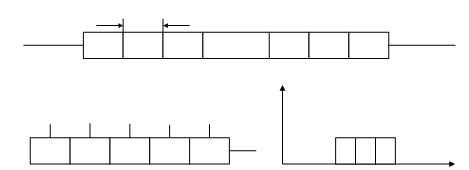## Applications of Shift Registers Assignment Help

Assignment Help: >> Sequential Circuits - Applications of Shift Registers

Applications of Shift Registers:

1. As a Delay Line : n number of D Flip Flops connected in series (SISO shift register) as illustrated in Figure (a) generates a propagation delay of n tp where tp is the propagation delay for one D Flip Flop and it may be utilized as delay line for applications in computers.

2. As a Sequence Generator: It may be realized by using a PISO shift register as depicted in Figure (b). Let us suppose that the waveform to be generated as shown in Figure (c) and its binary equivalent is 11100 where 1s represent positive voltage and 0 represents zero voltage. For this specific case, w may enter the data 11100 in parallel fashion at the preset terminals PR1 = 1, PR2 = 1, PR3 = 1, PR4 = 0 and PR5 = 0 keeping CLR = 1. The data will appear at the outputs of the Flip Flops and they may be taken out serially at the last output of Flip Flop by applying clock pulse.Figure: (a) SISO Shift Register as a Delay Line, (b) PISO Shift Register as a Sequence Generator, and (c) Waveform for Sequence Generator

3. As a Ring Counter : Assume the 3-bit shift register of Figure

Suppose that all the Flip Flops are cleared and then Flip Flop 1 is preset so that Q1 = 1 and Q2 = Q3 = 0. During the first clock, the data from Q1 shall be transferred to Q2. Therefore the data shall be progressively transferred around the Shift Register with each of clock pulse. If the last output Q3 and D1 are connected then the 1 state shall keep on circulating in the Flip Flops of the shift registers and this is called a Ring Counter and the count is read by noting which Flip Flop of the Shift Register is in state 1. For N Flip Flop based ring counters, it behaves as a Divide by N counter as there is one output pulse for each of clock pulse for N clock pulse.